Tableau Basics: An Article on Aggregate Functions in Calculated Fields

# Tableau Basics: An Article on Aggregate Functions in Calculated FieldsWant to be an expert in Tableau? Then you must start with the basics and learn them well. And to help you in your endeavors, we have created a blog series covering the fundamentals of Tableau. These articles are easy-to-follow and shall help you understand how and when to use the Calculated Field functions.

In this blog, we discuss Aggregate Functions. In Aggregate Functions, we group together multiple rows of values to form a single input value that is more meaningful, like a set or list. In order to access these functions select the option ‘Aggregate’ from the drop down list for functions in the ‘Create Calculated Filed’ window.

Now, let’s discuss the different types of Aggregate Functions one by one ad look into a few examples. A person having some experience in Excel will find these functions familiar.

##### ATTR(expression)

The ATTR function, short form for attribute, gives back a value when all rows have a single value. In case the values in the rows are different, the value ‘’*” is returned. It ignores null values. Example: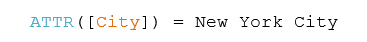##### AVG(expression)

The AVG function returns a value that is the average of all the values in a given expression. It is used only for numeric fields. Null values are not considered. Example: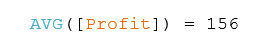##### COUNT(expression)

COUNT function returns the number of items present in a particular group. Null values are ignored. Example: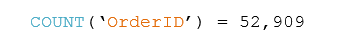##### COUNTD(expression)

COUNTD function returns distinct items in a group and counts them only once. Null values are ignored.

The function isn’t offered in certain types of workbooks, like the ones that were created prior to Tableau Desktop v8.2, workbooks where MS Excel or text files are used as sources of data, etc. Example: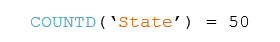##### MAX(expression)

A MAX function is used to obtain the maximum of two expressions for each record or the maximum of a single expression across all records. The two expressions must have the same type of argument. If either of the arguments is NULL, then NULL value is returned. Example: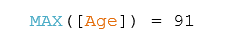##### MEDIAN(expression)

The median is the middle value of a sequence and the MEDIAN function is used to obtain the median for one particular expression. It only works for fields that are numeric. In case null values are present, they are ignored. Example: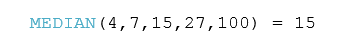##### MIN(expression)

The functionality of this function is similar to the MAX function. It is used to return the minimum of a single expression across all records or the minimum between two expressions for each record. If either of the two values is NULL, then a NULL value is returned. Like before, both the expressions need to have the same type of argument. Example: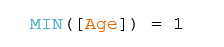##### PERCENTILE(expression, number)

A number between O and 1 is given and PERCENTILE function returns the percentile expression corresponding to that number. If 0.50 is given, then it returns the median number. Example: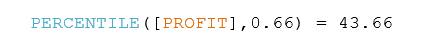##### STDEV(expression)

This is actually a statistical function and stands for standard deviation. STDEV function is used to obtain the statistical standard deviation for all values for a specific expression pertaining to the sample of a population.

##### STDEVP(expression)

The STDEVP function is similar to the STDEV function above, but it returns the statistical standard deviation for all the values in an expression that pertains to a biased population.

##### SUM(expression)

Simply put, this function adds up all the values in an expression. Example: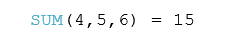##### VAR(expression)

VAR is another statistical function that returns the statistical variance for all the values in an expression pertaining to a sample of the population.

##### VARP(expression)

Similar to the function above, VARP function returns the statistical variance for all the values of an expression that pertains to the entire population.

Calculated Fields:

Calculated fields enable users to create more robust visualizations in Tableau. If you have missed our earlier blogs on Calculated Field functions, then visit the blog section of DexLab Analytics-we provide one of the best Tableau certifications in Delhi.

In order to be a Tableau expert, you need to enroll for comprehensive and well-structured Tableau BI training courses.